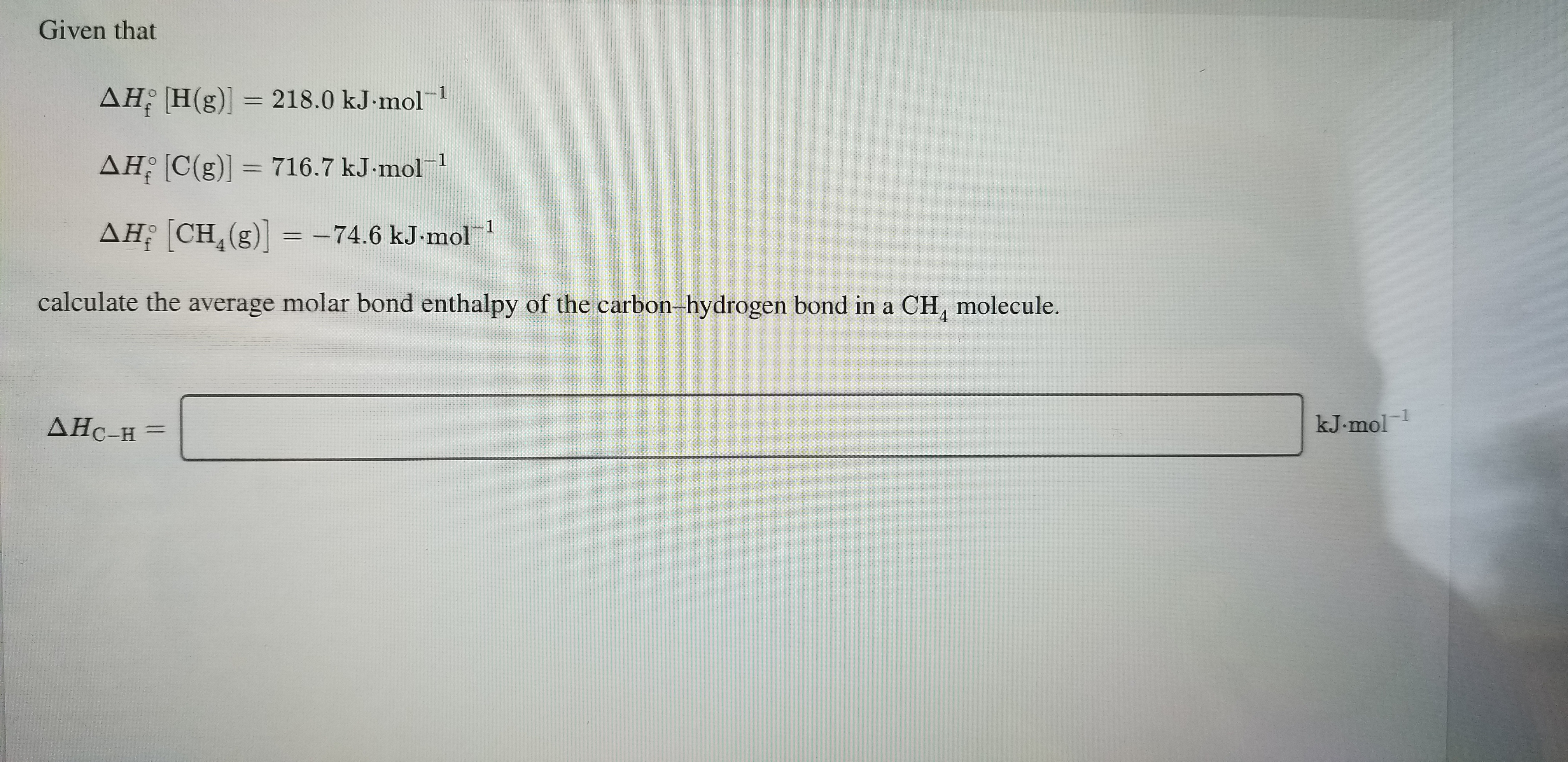# Given thatAH H(g)] =218.0 kJ mol1AH C(g)]= 716.7 kJ-mol1AH CH (g)= -74.6 kJ-mol 1calculate the average molar bond enthalpy of the carbon-hydrogen bond in a CH, molecule.1kJ.molДНС-н —

Question
1 viewshelp_outlineImage TranscriptioncloseGiven that AH H(g)] = 218.0 kJ mol1 AH C(g)]= 716.7 kJ-mol1 AH CH (g)= -74.6 kJ-mol 1 calculate the average molar bond enthalpy of the carbon-hydrogen bond in a CH, molecule. 1 kJ.mol ДНС-н — fullscreen
check_circle

Step 1

Given,

ΔHf [H(g)]= 218.0 kj/mol-1

ΔHf [C(g)]= 716.7 kJ/ mol-1

ΔHf [CH4(g)...

### Want to see the full answer?

See Solution

#### Want to see this answer and more?

Solutions are written by subject experts who are available 24/7. Questions are typically answered within 1 hour.*

See Solution
*Response times may vary by subject and question.
Tagged in

### General Chemistry